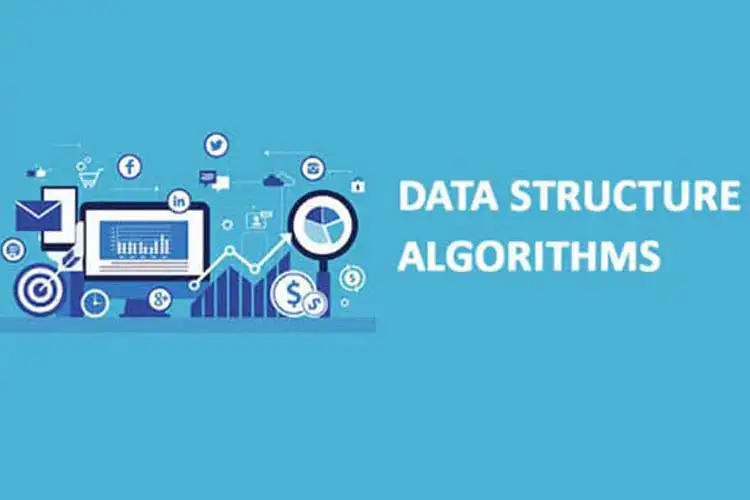# Data Structure and Algorithm

Goto "Data Science" Page### Data Structure and Algorithm

Best Programming Language Training Institute in Kolkata

A computer program is a collection of instructions to perform a specific task. For this, a computer program may need to store data, retrieve data, and perform computations on the data.

A data structure is a named location that can be used to store and organize data. And, an algorithm is a collection of steps to solve a particular problem. Learning data structures and algorithms allow us to write efficient and optimized computer programs.

Our DSA tutorial will guide you to learn different types of data structures and algorithms and their implementations in Python, C, C++, and Java.

****100% Job Assistance.****

Online Tution Available

## Our Successful Students

1 / 52 / 5Hariram Behera (B. Tech)
3 / 5Sayan Das (B. Tech)
4 / 5Samyabrata Chatterjee(MCA)
5 / 5Nirvik Verma (B.Tech)

### Why Learn Data Structur and Algorithm?

We offer a complete series of easy to follow DSA tutorials along with suitable examples. These tutorials are targeted for absolute beginners who want to dive into the field of computer programming.

• Write optimized and scalable code - Once you have knowledge about different data structures and algorithms, you can determine which data structure and algorithm to choose in various conditions.
• Effective use of time and memory - Having knowledge about data structures and algorithms will help you write codes that run faster and require less storage.
• Better job opportunities - Data structures and algorithms questions are frequently asked in job interviews of various organizations including Google, Facebook, and so on.

## Data Structure Course Details :

• → What is an algorithm?
• → Data Structure and Types
• → Why learn algorithms?
• → Asymptotic Notations
• → Master Theorem
• → Divide and Conquer Algorithm

• → Stack
• → Queue
• → Types of Queue
• → Circular Queue
• → Priority Queue
• → Deque

• → Types of Linked List
• → Hash Table
• → Heap Data Structure
• → Fibonacci Heap
• → Decrease Key and Delete node from Fibonacci Heap

• → Tree Data Structure
• → Tree Traversal
• → Binary Tree
• → Full Binary Tree
• → Perfect Binary Tree
• → Complete Binary Tree
• → Balanced Binary Tree
• → Binary Search Tree
• → AVL Tree

• → B Tree
• → Insertion into B-tree
• → Deletion from B-tree
• → B+ Tree
• → Insertion on a B+ Tree
• → Deletion from a B+ Tree
• → Red Black Tree
• → Insertion in Red Black Tree
• → Deletion from Red Black Tree

• → Graph Data Structure
• → Spanning Tree
• → Strongly Connected Components
• → DFS Algorithm
• → Bellman Ford's Algorithm

• → Bubble Sort
• → Selection Sort
• → Insertion Sort
• → Merge Sort
• → Quick Sort
• → Counting Sort
• → Bucket Sort
• → Heap Sort
• → Shell Sort
• → Linear Search
• → Binary Search

• → Greedy Algorithm
• → Ford-Fulkerson Algorithm
• → Dijkstra's Algorithm
• → Kruskal's Algorithm
• → Prim's Algorithm
• → Huffman Code

• → Dynamic Programming
• → Floyd Warshall Algorithm
• → Longest Common Subsequence

• → Backtracking Algorithm
• → Rabin-Karp Algorithm

 Minimum Eligibility: Computer Science Students COURSE FEE Rs. 10,000/- Course Duration: 2-3 Mode Of Training: Online and Offline

## Contact With Us

Mode Of Training: Classroom Online
Sex: Male Female